Question

# Provide the correct molecular geometry for (c), given the number of lone pairs and bonding groups...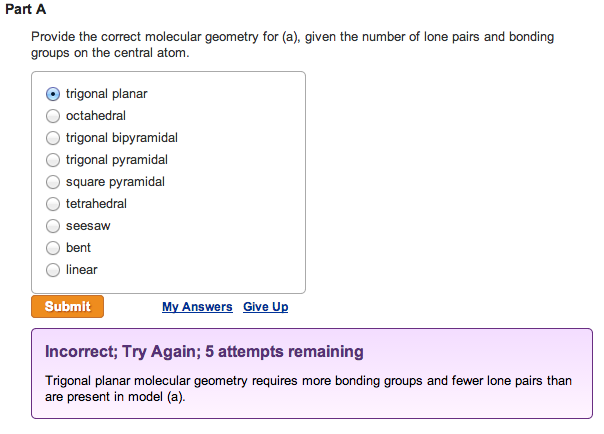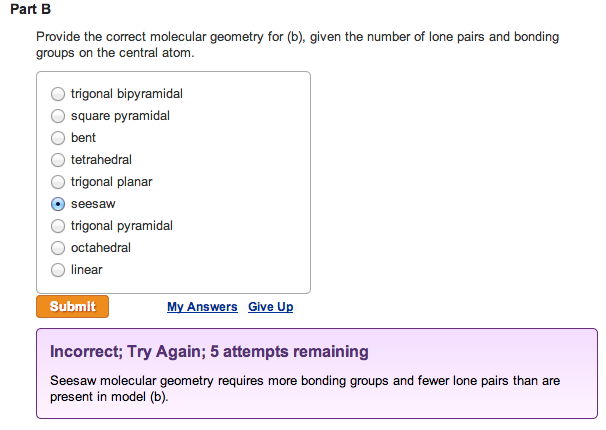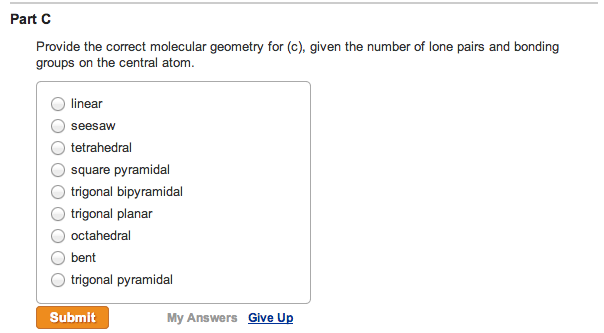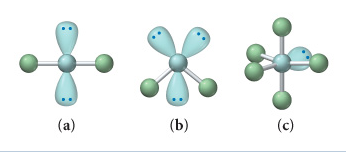Provide the correct molecular geometry for (a), given the number of lone pairs and bonding groups on the central atom.

trigonal planar  octahedral  trigonal bipyramidal  trigonal pyramidal  square pyramidal  tetrahedral  seesaw  bent  linear

Provide the correct molecular geometry for (b), given the number of lone pairs and bonding groups on the central atom.

trigonal bipyramidal  square pyramidal  bent  tetrahedral  trigonal planar  seesaw  trigonal pyramidal  octahedral  linear

Provide the correct molecular geometry for (c), given the number of lone pairs and bonding groups on the central atom.

linear  seesaw  tetrahedral  square pyramidal  trigonal bipyramidal  trigonal planar  octahedral  bent  trigonal pyramidal

Concepts and reason

The concept used here is VSEPR theory. The theory is used to predict the geometry of most polyatomic molecules or ions by considering the number of electron pairs around the central atom. Each group around the central atom is designated as a bond pair or lone pair. The repulsion between the valence electron pairs on the central atom determines the geometry of the molecule.

Fundamentals

For predicting the geometry of molecules, the number of electron pairs (bonding and lone pairs) is counted. Lone pairs repel more than the bonding pairs. The number of lone pairs determines the correct molecular geometry of a molecule.

Part A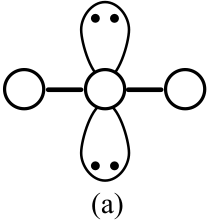Number of valence electrons pairs (bond pairs + lone pairs) on the central atom is equal to 4. The number of bond pairs is 2 and number of lone pairs is also 2. The number of valence electrons pairs is 4. Thus, geometry of this structure cannot be octahedral, trigonal bipyramidal, trigonal pyramidal, square pyramidal, seesaw or linear.

Part A

The four electron pairs are spread tetrahedrally around the central atom. But, the presence of two bond pairs and two lone pairs causes bond pair-lone pair repulsion, which results in bent structure.

Part B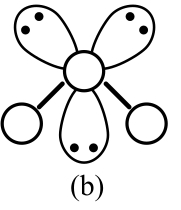Number of valence electrons pairs (bond pairs + lone pairs) on the central atom is 5. The number of bond pair and lone pair are 2 and 3 respectively. The number of valence electrons are 5. Thus, geometry cannot be square pyramidal, bent, tetrahedral, seesaw, octahedral , trigonal planar or trigonal pyramidal.

Part B

The five electron pairs are distributed in trigonal bipyramidal arrangement. To minimize the repulsion, the three lone pairs are arranged in equatorial position. This results in linear molecular geometry.

Part C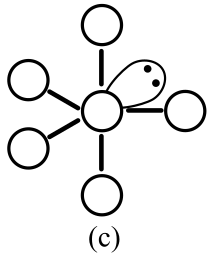Number of valence electrons pairs (bond pairs + lone pairs) on the central atom is 6. The number of bonding pair and lone pairs are 5 and 1 respectively. Due to 6 valence electrons, geometry cannot be bent, tetrahedral, seesaw, linear, trigonal planar or trigonal pyramidal.

The six electron pairs give octahedral structure. Presence of one lone pair results in square pyramidal geometry.

Ans: Part A

The correct molecular geometry for (a) is bent.

Part B

The correct molecular geometry for (b) is linear.

Part C

The correct molecular geometry for (c) is square pyramidal.

#### Earn Coins

Coins can be redeemed for fabulous gifts.

Similar Homework Help Questions
• ### Number 1-6 1. What is the molecular geometry of SBr4? A) seesaw B) square planar C)...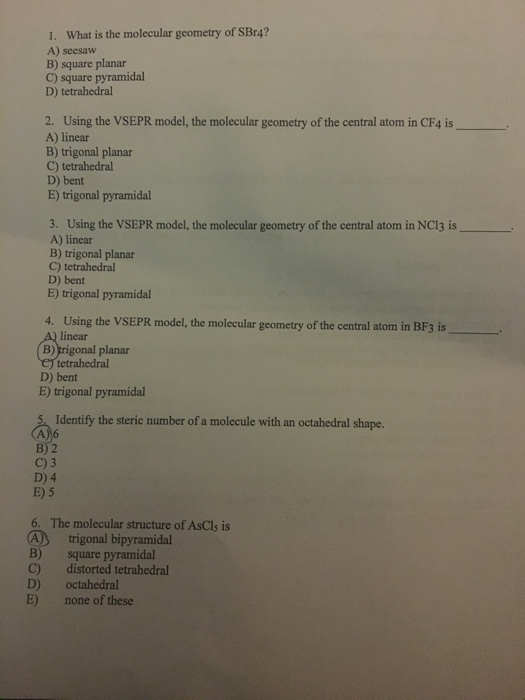Number 1-6 1. What is the molecular geometry of SBr4? A) seesaw B) square planar C) square pyramidal D) tetrahedral 2. Using the VSEPR model, the molecular geometry of the central atom in CF4 is A) linear B) trigonal planar C) tetrahedral D) bent E) trigonal pyramidal 3. Using the VSEPR model, the molecular geometry of the central atom in NC13 is A) linear B) trigonal planar C) tetrahedral D) bent E) trigonal pyramidal 4. Using the VSEPR model, the...

• ### Draw the Lewis structure of CIBr, showing all lone pairs. Identify the molecular geometry of CIBry...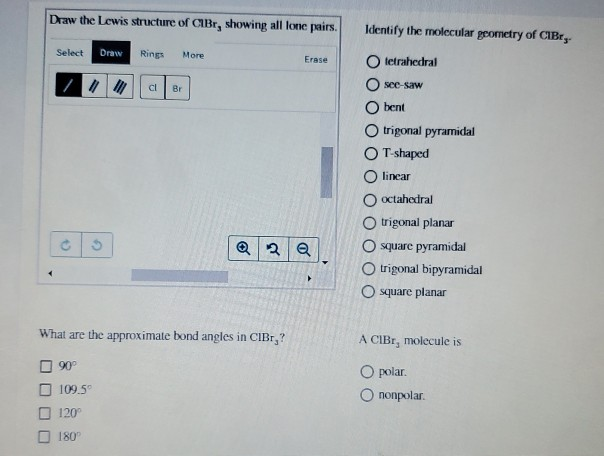Draw the Lewis structure of CIBr, showing all lone pairs. Identify the molecular geometry of CIBry Select Draw Rings More Erase tetrahedral see-saw bent trigonal pyramidal T-shaped O linear octahedral trigonal planar O square pyramidal trigonal bipyramidal square planar What are the approximate bond angles in CIBr,? A CIBr, molecule is O polar. Ononpolar. 90° 109.5 120 180

• ### According to VSEPR theory, XeO3 has how many regions of electron density around the central atom...

According to VSEPR theory, XeO3 has how many regions of electron density around the central atom (how many electron groups?) (enter a number from 1 to 6) ? XeO3 will have (linear, trigonal planar, tetrahedral, trigonal pyramidal, bent, trigonal bipyramidal, seesaw, T-shaped, octahedral, square pyramidal, square planar) electronic geometry, and (linear, trigonal planar, tetrahedral, trigonal pyramidal, bent, trigonal bipyramidal, seesaw, T-shaped, octahedral, square pyramidal, square planar) molecular geometry. Draw the best Lewis structure using formal charge information. How many single...

• ### Draw the Lewis structure of XeCl. Include all lone pairs. Identify the molecular geometry of Xeci,...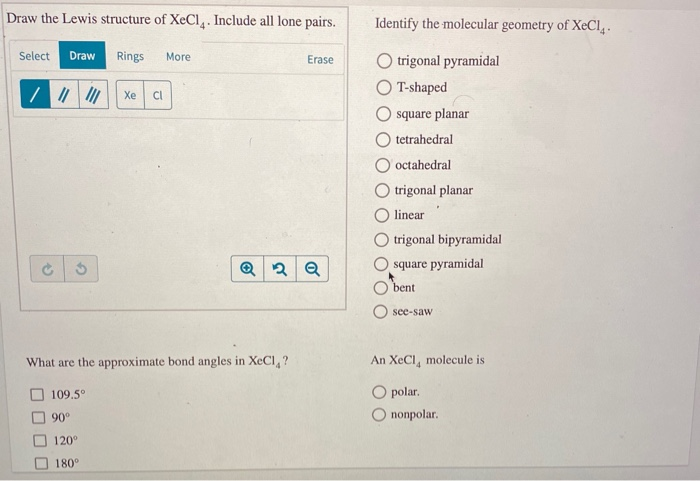Draw the Lewis structure of XeCl. Include all lone pairs. Identify the molecular geometry of Xeci, Select Draw Rings More Erase trigonal pyramidal T-shaped square planar O tetrahedral O octahedral trigonal planar linear trigonal bipyramidal square pyramidal bent O see-saw What are the approximate bond angles in XeCl,? An XeCl, molecule is O polar O nonpolar. 109.5° 90° 120° 180°

• ### Using VSEPR theory, a molecule with the general formula AX E# (A is the central atom,...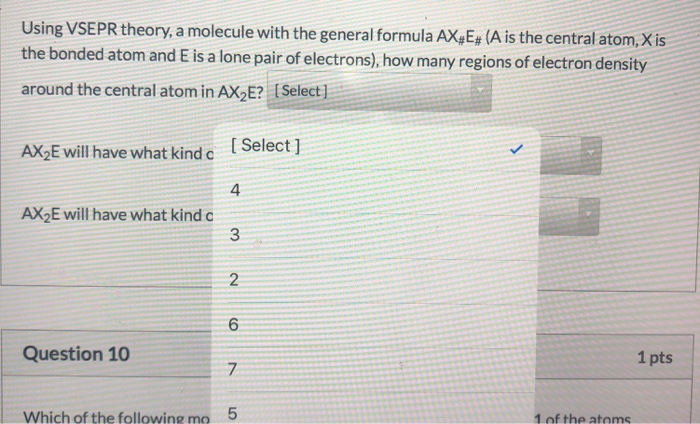Using VSEPR theory, a molecule with the general formula AX E# (A is the central atom, Xis the bonded atom and E is a lone pair of electrons), how many regions of electron density around the central atom in AX E? [Select] AX2E will have what kind c [Select] AX2E will have what kind a w N o Question 10 1 pts V Which of the following mo 5 1 of the atoms 5:35 PM Using VSEPR theory, a molecule...

• ### Identify the type of molecular geometry of six electron pairs about a central atom (in which...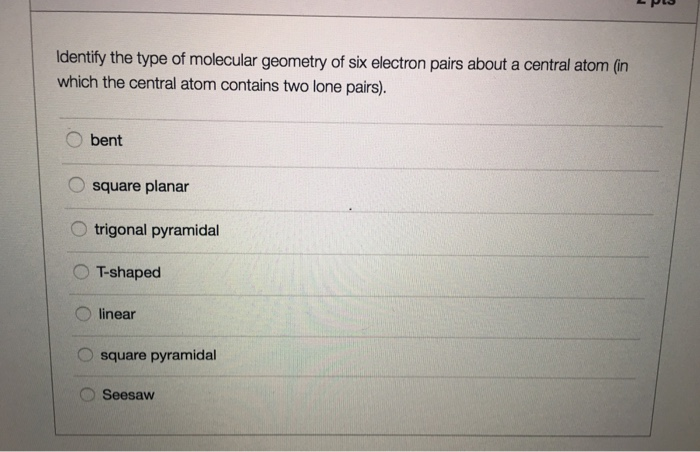Identify the type of molecular geometry of six electron pairs about a central atom (in which the central atom contains two lone pairs). bent square planar trigonal pyramidal T-shaped linear square pyramidal Seesaw

• ### VSEPR theory predicts which shape for the molecular structure of the following molecules or molecular ions?...

VSEPR theory predicts which shape for the molecular structure of the following molecules or molecular ions? (i.e. the arrangement of only the bonded atoms about the central atom) bent (or angular) linear octahedral see-saw square planar square pyramidal tetrahedral trigonal bipyramidal trigonal planar trigonal pyramidal T-shaped  ICl3 molecule    (The central atom is I.) bent (or angular) linear octahedral see-saw square planar square pyramidal tetrahedral trigonal bipyramidal trigonal planar trigonal pyramidal T-shaped  IO4- ion    (The central atom is I.) bent (or angular) linear...

• ### Please answer correctly I'll give best rating if you do correct one Electron Geomctry Name Choices:...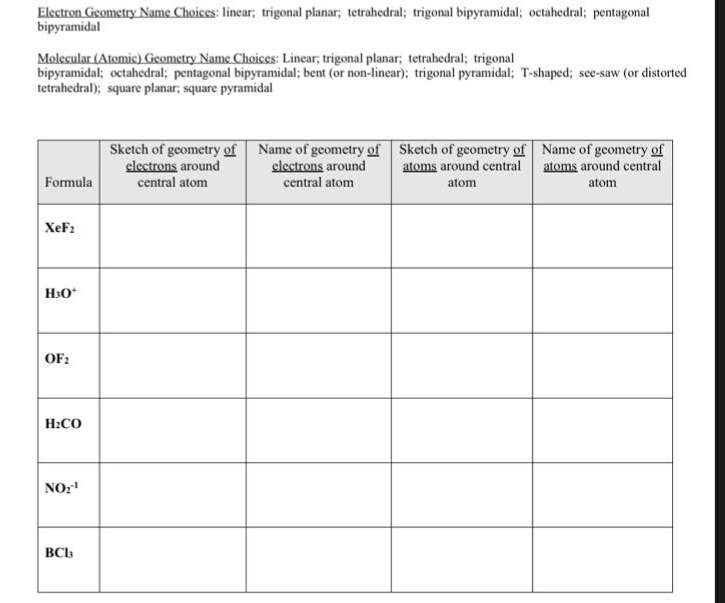Please answer correctly I'll give best rating if you do correct one Electron Geomctry Name Choices: linear; trigonal planar; tetrahedral; trigonal bipyramidal; octahedral; pentagonal bipyramidal Molecular (Atomic) Geometry Name Choices: Linear, trigonal planar; tetrahedral; trigonal bipyramidal; octahedral; pentagonal bipyramidal; bent (or non-linear); trigonal pyramidal: T-shaped; see-saw (or distorted tetrahedral); square planar, square pyramidal Sketch of geometry of Name of geometry of Sketch of geometry of Name of geometry of clectrons around central atom clectrons around atoms around central atoms around...

• ### When the central atom has three bonded groups and one lone pair, the molecular geometry (shape)...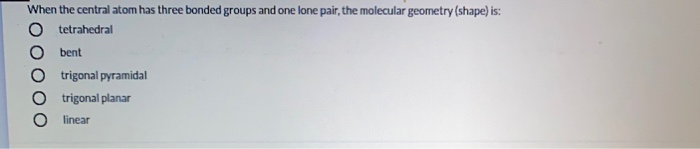When the central atom has three bonded groups and one lone pair, the molecular geometry (shape) is: tetrahedral bent trigonal pyramidal trigonal planar linear

• ### Number of Electron Groups Ideal Bond Angles VSEPR Molecular Shape Answer Bank bent Bonded Atoms/ Lone...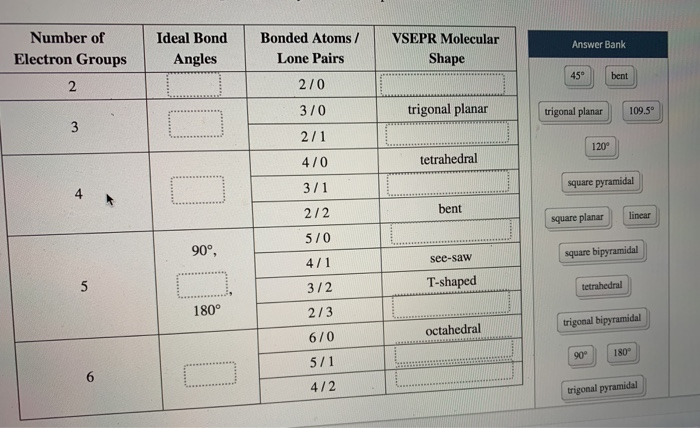Number of Electron Groups Ideal Bond Angles VSEPR Molecular Shape Answer Bank bent Bonded Atoms/ Lone Pairs 270 370 2/1 trigonal planar trigonal planar 109.5 120 470 tetrahedral 3/1 square pyramidal bent square planar linear 90° square bipyramidal 2/2 570 4/1 3/2 2/3 see-saw T-shaped tetrahedral trigonal bipyramidal octahedral 6/0 5/1 4/2 trigonal pyramidal Which of the substances has polar interactions (dipole-dipole forces) between molecules? HF Cci, CHCI, so, tu a m carrers privacy polic